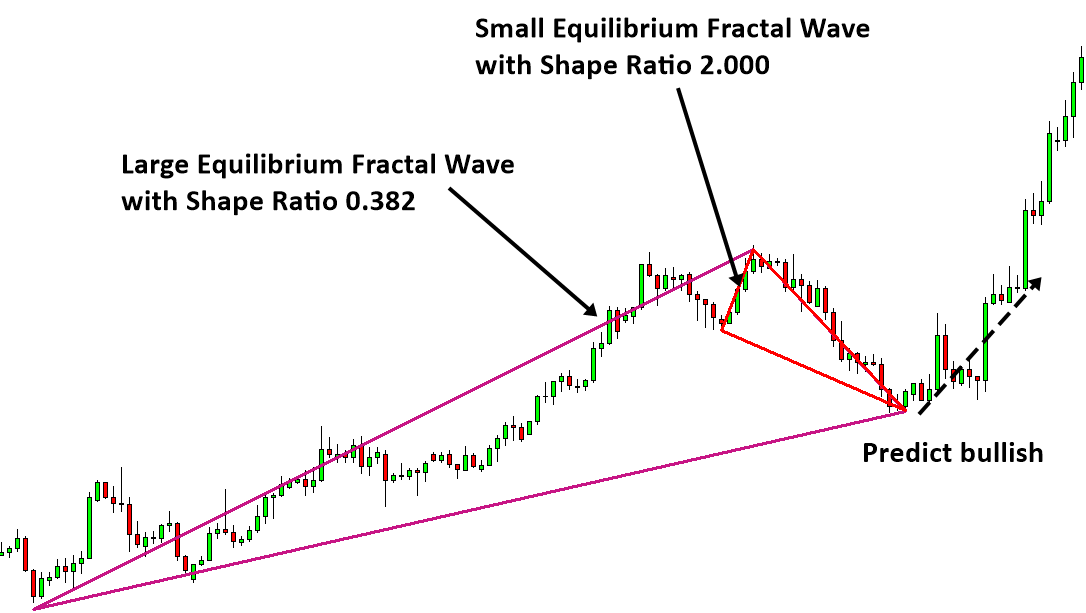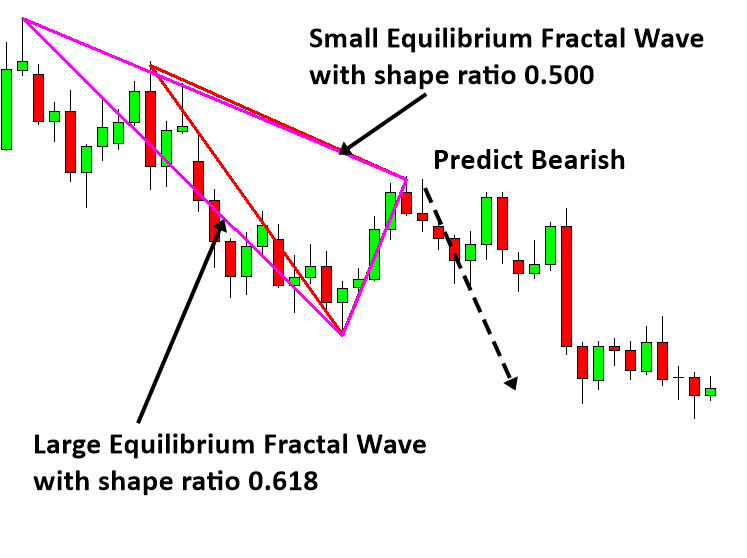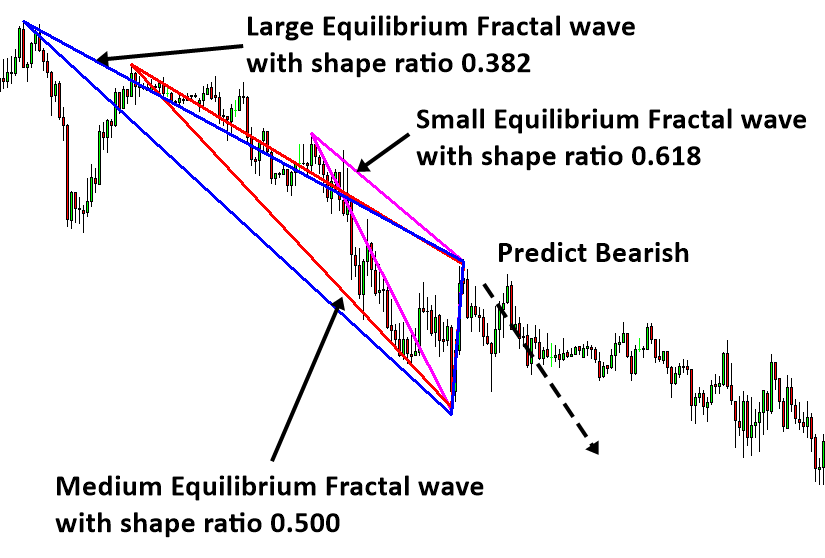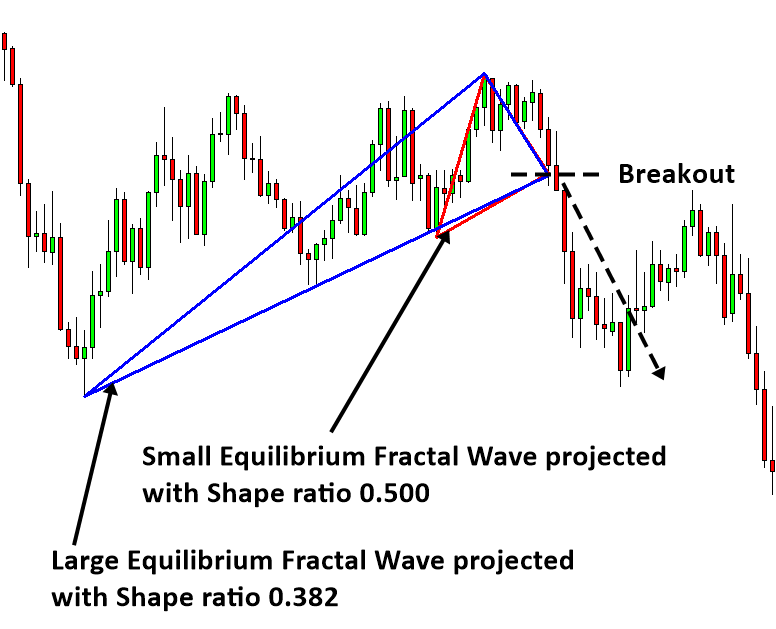# Superimposed Equilibrium Fractal Waves

Third characteristic of equilibrium fractal wave is that smaller equilibrium fractal waves can combine to form a bigger equilibrium fractal wave (superimposed). This third characteristic is often used by a professional trader to improve the predictability of the financial market. For example, instead of puzzling with a set of small equilibrium fractal waves, it is much more accurate to puzzle with both small and big equilibrium fractal waves together to predict the market direction. Now we show some examples of our reversal prediction using this superimposed equilibrium fractal waves. In Figure 3-23 and Figure 3-24, a large Equilibrium fractal wave is overlapping with a small equilibrium fractal wave. We can make the bullish reversal prediction after the last candle is completed. Making the prediction based on the two Equilibrium fractal waves can increase the probability of winning marginally over just using one equilibrium fractal wave.Figure 3-23: Superimposed Equilibrium fractal wave for bullish reversal trading setup (EURUSD D1).Figure 3-24: Superimposed Equilibrium fractal wave for bullish reversal trading setup (EURUSD H1).

Likewise, we can use these superimposed equilibrium fractal waves to make the bearish reversal prediction too as shown in Figure 3-25 and Figure 3-26. You can even use three or four equilibrium fractal waves for your prediction. However, the superimposed patterns with three or four equilibrium fractal waves are rare. If they do appears then they can provide a good trading opportunity.Figure 3-25: Superimposed Equilibrium fractal wave for bearish reversal trading setup (EURUSD H1).Figure 3-26: Superimposed Equilibrium fractal wave for bearish reversal trading setup (EURUSD M15).

Trader should not assume that he could predict the reversal every time. Increased probability does not mean that you are guaranteed to be right every time. Based on the second characteristic of Equilibrium fractal wave, extension, the price can still penetrate these superimposed equilibrium fractal waves for the breakout opportunities. When they do, they can penetrate the superimposed level with even higher energy than the level projected by single equilibrium fractal wave. To trade with the superimposed level, you have to project the shape ratios from several equilibrium fractal waves in advance. Once you can identify the overlapping level between several Equilibrium fractal waves, you can trade for the breakout opportunity. When you trade the breakout opportunity, you can use the idea of trigger level we taught you before in the support and resistance trading.Figure 3-27: Superimposed projection for bullish breakout trading setup (EURUSD D1).Figure 3-28: Superimposed projection for bearish breakout trading setup (USDJPY H1).

This article is the part taken from the draft version of the Book: Scientific Guide to Price Action and Pattern Trading (Wisdom of Trend, Cycle, and Fractal Wave). Full version of the book can be found from the link below: